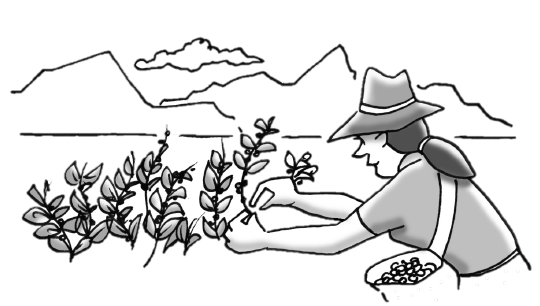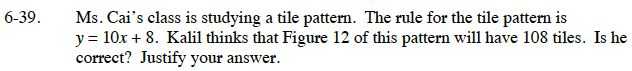### Home > CC3 > Chapter 6 > Lesson 6.1.4 > Problem6-39

6-39.Ms. Cai’s class is studying a tile pattern. The rule for the tile pattern is y = 10x + 8. Kalil thinks that Figure 12 of this pattern will have 108 tiles. Is he correct? Justify your answer. Homework Help ✎y = # of tiles
x = Figure #

Substitute the information from the problem into the equation
y = 10x + 8.

Kalil is wrong.

108 = 10(12) + 8
108 = 120 + 8

108 ≠ 128 tiles